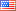# Revision history [back]

### How to reference algebra monomials?

Hello. My questions are relatively easy to phrase, but first, some background.

If I have an algebra like H = IwahoriHeckeAlgebraT("A3",1,prefix = "s"), and I ask for H(H.basis().keys()) I get s1, which is the correct ewaulr and the same result as H.monomial(H.basis().keys()). However, with an algebra such as A = CombinatorialFreeModule(QQ, ['a','b','c']), A(A.basis().keys()) returns an error, whereas A.monomial(A.basis().keys()) returns B[b], which is the correct answer.

So, my question is, what is the difference between calling an Algebra Object (like A or H from above) versus calling that Algebra Object's monomial method? Furthermore, if they are different, (which they appear to be,) what method is A(x) or H(x) calling? Finally, is there any documentation on this A(x) method? Calling the documentation from the notebook interface gets me the docstring for the class.2 No.2 RevisionJohn Palmieri5718 ●18 ●51 ●126 http://www.math.washin...

### How to reference algebra monomials?

Hello. My questions are relatively easy to phrase, but first, some background.

If I have an algebra like

H = IwahoriHeckeAlgebraT("A3",1,prefix = "s"), "s")


and I ask for H(H.basis().keys()) H(H.basis().keys()) I get s1, which is the correct ewaulr and the same result as H.monomial(H.basis().keys()). H.monomial(H.basis().keys()). However, with an algebra such as as

A = CombinatorialFreeModule(QQ, ['a','b','c']), A(A.basis().keys()) ['a','b','c'])


A(A.basis().keys()) returns an error, whereas A.monomial(A.basis().keys()) A.monomial(A.basis().keys()) returns B[b], which is the correct answer.

So, my question is, what is the difference between calling an Algebra Object (like A or H from above) versus calling that Algebra Object's monomial method? Furthermore, if they are different, (which they appear to be,) what method is A(x) or H(x) calling? Finally, is there any documentation on this A(x) method? Calling the documentation from the notebook interface gets me the docstring for the class.3 retaggedFrédéricC3139 ●3 ●33 ●61

### How to reference algebra monomials?

Hello. My questions are relatively easy to phrase, but first, some background.

If I have an algebra like

H = IwahoriHeckeAlgebraT("A3",1,prefix = "s")


and I ask for H(H.basis().keys()) I get s1, which is the correct ewaulr and the same result as H.monomial(H.basis().keys()). However, with an algebra such as

A = CombinatorialFreeModule(QQ, ['a','b','c'])


A(A.basis().keys()) returns an error, whereas A.monomial(A.basis().keys()) returns B[b], which is the correct answer.

So, my question is, what is the difference between calling an Algebra Object (like A or H from above) versus calling that Algebra Object's monomial method? Furthermore, if they are different, (which they appear to be,) what method is A(x) or H(x) calling? Finally, is there any documentation on this A(x) method? Calling the documentation from the notebook interface gets me the docstring for the class.